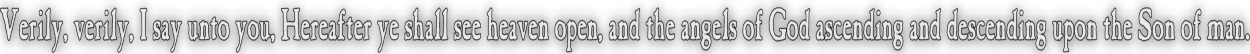None:
Polyps:
Strongs:MetaphysicsReasoning As A Universal Being We will introduce a "universal being" (i.e. as if the Holy Spirit) which we will call "U" and will assume that U may reason so perfectly that anything true on the consistency of existence of another being may be reasoned to another. I.e. if P(cogG(¬x)) then we immediately find P(cogU(¬x)) => P(cogx(¬x)). Likewise ¬P(cogG(¬x)) => ¬P(cogU(¬x)) => ¬P(cogx(¬x)) and x considers itself 'necessary'. We assume that U not only reasons perfectly but in a manner common to both perfection (God) and those beings that are able to conceive of a necessary being. We have already had a brush with "U" in the treatment of the trinity transforming perfectly. We said that: (H~G&p) & (G&p~H) >= super(H~G & G~H) using our prior equivalence relation '~'. Whereas we can now state (cogH(G&p) & cogG&p(H)) >= (cogG(H) & (cogH(G)) Or we may state that if it is positive (i.e. Pos(...)) that cogH(G&p) then Pos(cogU(G&p)) which results in Pos(cogG(G&p)) => G&p Likewise Pos(cogH(G&¬p)) then Pos(cogU(G&¬p)) which results in Pos(cogG(G&¬p)) => G&¬p We should note that the "cog" operator has two distinct parts: There is the suffix 'x' in cogx(y) as well as the operand 'y'. "x" is considered a concrete being, whereas "y" is an abstraction. Alternatively consider the suffix as an "earthly" individual and the operand as "heavenly". We will state that from the existence of U that 'x' in cogx(G) may conceive of God (G) consistently if and only if U may do so also. Thus (cogx(G)<=> cogU(G)) => cogG(G) => N(G) (i.e. we infer from Hartshorne's assumption that "perfection is possible".) So that a consistently conceived perfect being must exist necessarily if at all: Or that it is universally correct for "G~x", which entails or requires that cogG(x) is consistent in that the "image" of God reciprocated by 'x' in the definition of "being" i.e. cogG(x) is consistent with the reciprocated belief of the nature of 'G' Himself. "x~G" => "U~G" if G is consistent. Thus G=>N(G) from Hartshorne's assumption with Anselm's principle. Then by reflexivity from the definition of '~', we have "G~U" and "U~x" => G~x (transitivity) Which requires symmetry also from the definition of '~' leading to "G~x" <=> "x~G" or "G~y" universally for all 'y' including U. What objections may we make? U~G is consistent is part and parcel with G~U, for "U is a being" to God that engenders perfect knowledge of the consistency of 'G'. U~x is consistent in that U may conceive of any "being" 'x' ('x' likewise able to reason) that with reason may in itself be consistent as with itself (whether x~x or x~U). We make no assumption as to whether U is necessary or contingent. Likewise it follows from U~x that both G~x and x~x (and y~x) for any G, x and y. There is no assumption that "U" is "impossible", neither is U's existence able to be contradicted. The result then that G~G is completely consistent carries from the result that G~x implies in itself that x~G may be done so just as consistently, and if it is possible for U~G to show reflexivity then it is true that G is consistent for all 'x' that hold true to that "one correct God held by U". We would expect the transitivity of G~U and U~x to imply that G~x is consistent with G Himself. We may substitute G or 'x' for U and have a valid result from our definition of '~'. There is no contradiction in the definition of "being" whilst using our relation '~'. Continue To Next Page Return To Section Start Return To Previous Page'